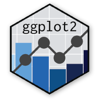Show Sidebar Hide Sidebar# geom_rect in ggplot2

How to make a timeline using geom_rect.

### New to Plotly?

Plotly's R library is free and open source!
You can set up Plotly to work in online or offline mode.
We also have a quick-reference cheatsheet (new!) to help you get started!

### Version Check

Version 4 of Plotly's R package is now available!
Check out this post for more information on breaking changes and new features available in this version.

library(plotly)
packageVersion('plotly')

##  '4.9.1'


### geom_rect with a line graph

geom_rect is defined by its four sides (xmin, xmax, ymin, ymax), which are all included in the dataset. Fill refers to the colour of the rectangle, colour refers to the border, and size refers to the border width.

This line graph shows the unemployment number in the United States every month, beginning in July 1967. (This dataset comes with R.) We use geom_rect to shade the background according to the President's party. An important note: the President does not control economic policy, something that this graph hopefully makes apparent. This is for illutrustive purposes, using a simple case and a default dataset.

library(plotly)

library(dplyr)

df <- data.frame(name = c("Nixon", "Ford", "Carter", "Reagan", "Bush", "Clinton", "Bush", "Obama"),
start = as.Date(c("1969-01-20", "1974-08-09", "1977-01-20", "1981-01-20",
"1989-01-20", "1993-01-20", "2001-01-20", "2009-01-20")),
end = as.Date(c("1974-08-09", "1977-01-20", "1981-01-20", "1989-01-20",
"1993-01-20", "2001-01-20", "2009-01-20", "2017-01-20")),
party = c("R", "R", "D", "R", "R", "D", "R", "D"),
stringsAsFactors = FALSE) %>%
mutate(median_x = start + floor((end-start)/2))

p <- ggplot(economics, aes(x=date,y=unemploy)) +
geom_rect(data=df, aes(NULL,NULL,xmin=start,xmax=end,fill=party),
ymin=0,ymax=16000, colour="white", size=0.5, alpha=0.2) +
scale_fill_manual(values=c("R" = "red", "D" = "blue")) +
geom_line() +
geom_text(data=df,aes(x=median_x,y=3000,label=name), size=3) +
labs(title = "Unemmployment numbers since 1967",
y = "No. unemployed (x 1000)")
p <- ggplotly(p)

p


### A Timeline Using geom_rect

geom_rect could also be the "main course" rather than just the background, for example by making a timeline. Here are the leaders of the current European Union member countries, since 2000. Data comes from ParlGov (which is where the parties' left-right scores come from, so please direct your complaints elsewhere). The LaCroixColoR package is used and can be installed using the instructions in the GitHub Readme.

library(plotly)
library(LaCroixColoR)
library(dplyr)

stringsAsFactors = FALSE) %>%
mutate(stint_start = as.Date(stint_start),
stint_end = as.Date(stint_end),
median_x = as.Date(median_x),
left_right = as.character(left_right))

p <- ggplot(european_leaders, aes(xmin=stint_start, xmax=stint_end, ymin=vert_min, ymax=vert_max, fill=left_right)) +
geom_rect(colour="white", size=0.1) +
geom_text(aes(x=median_x, y=median_y, size=size, label=pm_name, #all names of separate variables
text=paste(party_name_english, " (",country_name,")", sep=""))) +
scale_fill_manual(values=lacroix_palette("PassionFruit",type = "continuous", n=8)) + #matches left/right colours
labs(title = "Timeline of European leaders since 2000",
x = "year",
y = "",
fill = "Party's \nleft-right \nscore",
size = NULL) +
theme(axis.text.y = element_blank(), #y-axis doesn't have actual values; no need for labels
axis.ticks.y = element_blank())
p <- ggplotly(p, tooltip = c("label", "text")) #how to select what shows on the tooltip

p